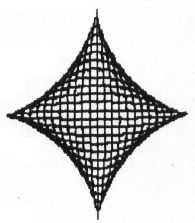`COMPUTE! ISSUE 30 / NOVEMBER 1982 / PAGE 14`

 VIC Superexpander Graphics This program will let you transfer screens of graphics created with the VIC Superexpander to the 1515 printer. It works best in modes two and three and, while it does give 100% resolution, it takes just over ten minutes per screen.```10 GRAPHIC2 : COLOR 0, 0, 5, 5 : FORU = 0T0500STEP40 : CIRCLE2, 511, 511, U, 500-U : NEXT 20 DIMAS\$ (19) 30 Y = 4096 : OPEN1, 4 40 FORE = 0T0154STEP7 : D = -1 50 FORZ = 0TO190STEP10 : D = +1 60 W = Y + (Z*16) + E 70 FORF = 0T06 80 A% (F) = PEEK (W + F) 90 NEXTF 95 FORT = 0TO9 : B% (T) = 0: NEXTT 100 S = 256:FORL = 0TO7 : S = S/2: FORI = 0TO6 110 IF (A% (I) ANDS) = STHENB% (L) = B% (L) + (2 ↑ I) 120 NEXTI : NEXTL 130 FORT = 0TO7 : IFB% (F) <128THENB% (F) =B% (F) + 128 140 A\$ (D) =A\$ (D) + CHR\$ (B% (F)) : NEXTF 150 IFD<19 THENNEXTZ 160 FORF = 0TO18 170 PRINT # 1, CHR\$ (8) A\$ (F); 180 A\$ (F) "" : NEXTF 190 PRINT #1, CHR\$ (8) A\$ (19) 200 A\$ (19) = "" : R = FRE (0) 210 NEXTE 220 PRINT # 1, CHR\$ (15) : CLOSE1 ``` Richard Hollern